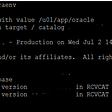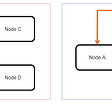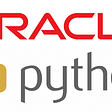# Background

Let us start with two examples to understand how does the rank calculation work on PostgreSQL.

# Example 1

The following two records are available in the database.

`"I want a dog"  // 狗  "I want a chihuahua"  // 吉娃娃狗`

Use the following query condition and run a query.

`"dog|chihuahua"`

Clearly, both records match. However, the question here is which one should be displayed at the top?

# Example 2

Now, search for “dog|chihuahua” in the search engine and see which record will be displayed at the top? Skip to the second page to avoid advertisements.

As shown in the preceding snapshot, records about Chihuahua are displayed first. You may ask, why?

It is due to the term frequency-inverse document frequency (TF-IDF) algorithm. In a global text, the occurrence frequency of the word dog is higher than that of Chihuahua. Therefore, according to the inverse document frequency (IDF) algorithm, the IDF (log (total number of texts/number of texts containing the word)) of the word ‘dog’ is lower than that of ‘Chihuahua’.

However, in case if TF is the same, the size of TF × IDF depends on the size of IDF. PostgreSQL databases support full-text search. But, do they support TF-IDF by default?

# PostgreSQL Databases Do Not Use IDF to Calculate the Rank by Default

According to the code, the ts_rank and ts_rank_cd functions of PostgreSQL do not take IDF into consideration.

`Within the ts_rank function, there is no native method to rank results using their global (corpus) frequency. The rank algorithm does however rank based on frequency within the document:    http://www.postgresql.org/docs/9.3/static/textsearch-controls.html    So if I search for "dog|chihuahua" the following two documents would have the same rank despite the relatively lower frequency of the word "chihuahua":    "I want a dog"  // 狗  "I want a chihuahua"  // 奇瓦瓦狗    However, the following line would get ranked higher than the previous two lines above, because it contains the stemmed token "dog" twice in the document:    "dog lovers have an average of 1.5 dogs"    In short: higher term frequency(TF) within the document results in a higher rank, but a lower term frequency in the corpus has no impact.    One caveat: the text search does ignore stop-words, so you will not match on ultra high frequency words like "the","a","of","for" etc (assuming you have correctly set your language)  Postgres does not use TF-IDF as a similarity measure among documents.    ts_rank is higher if a document contains query terms more frequently. It does not take into account the global frequency of the term.    ts_rank_cd is higher if a document contains query terms closer together and more frequently. It does not take into account the global frequency of the term.    There is an extension from the text search creators called smlar, that lets you calculate the similarity between arrays using TF-IDF.     It also lets you turn tsvectors into arrays, and supports fast indexing.`

PostgreSQL’s built-in rank algorithm does not care about the IDF, instead only calculates the word frequency in the current text.

Currently, PostgreSQL uses ts_rank and ts_rank_cd to calculate the correlation between tsquery and tsvector. For more details about the algorithm, refer to the previous blog in this series Keyword Analysis with PostgreSQL: Cosine and Linear Correlation Algorithms for Text Analysis.

# Introduction to the smlar Plug-in

The smlar plug-in supports multiple similarity calculation formulas (algorithms), including cosine (default), TF-IDF, and overlap. It also allows you to customize a formula or function to calculate the similarity.

# Function API

1. Calculate the similarity between arrays.

`float4 smlar(anyarray, anyarray)  - computes similary of two arrays. Arrays should be the same type.`

2. Calculate the similarity between custom composite arrays (elements and weights). You can select to calculate all elements or only the overlapped elements. When the weights are different, the similarity result is different, for example, in the cosine algorithm.

Alternatively, you can take the array as a weighted array that contains TD-IDF, such as [(‘China’, 0.1), (‘Japan ‘, 0.1), (‘pirate’, 0.9)]. It is useful for text similarity analysis.

`float4 smlar(anyarray, anyarray, bool useIntersect)      -  computes similary of two arrays of composite types. Composite type looks like:      CREATE TYPE type_name AS (element_name anytype, weight_name FLOAT4);         useIntersect option points to use only intersected elements in denominator         see an exmaples in sql/composite_int4.sql or sql/composite_text.sql`

Consider the following example:

`postgres=# create type tp as (c1 text, c2 float4);CREATE TYPEpostgres=# select smlar(array[('你好',2.1), ('中国',1.1)]::_tp, array[('你好',2.1), ('中国',1.1),('china',1)]::_tp);  smlar   ---------- 0.816497(1 row)postgres=# select smlar(array[('你好',2.1), ('中国',1.1)]::_tp, array[('你好',2.1), ('中国',1.1),('china',1)]::_tp,true); smlar -------     1(1 row)postgres=# select smlar(array[('你好',2.1), ('中国',1.1)]::_tp, array[('你好',2.2), ('中国',1.1),('china',1)]::_tp,true);  smlar   ---------- 0.999822(1 row)与顺序无关postgres=# select smlar(array[('你好',2.1), ('中国',1.1)]::_tp, array[('中国',1.1),('你好',2.2),('china',1)]::_tp,true);  smlar   ---------- 0.999822(1 row)`

Note: GiST doesn’t support composite weighted type.

`elog(ERROR, "GiST  doesn't support composite (weighted) type");`

3. Use a custom formula that contains three variables, namely, N.i, N.a, and N.b, to calculate the similarity between arrays. You can also customize the algorithm.

`float4 smlar( anyarray a, anyarray b, text formula );      - computes similary of two arrays by given formula, arrays should       be the same type.       Predefined variables in formula:        N.i    - number of common elements in both array (intersection)        N.a   - number of uniqueelements in first array        N.b   - number of uniqueelements in second array      Example:      smlar('{1,4,6}'::int[], '{5,4,6}' )      smlar('{1,4,6}'::int[], '{5,4,6}', 'N.i / sqrt(N.a * N.b)' )      That calls are equivalent.`

# Operator API

Determine whether both arrays are similar. Note, when the similarity value is greater than the threshold, the threshold is specified by the smlar.threshold parameter).

`anyarray % anyarray      - returns true if similarity of that arrays is greater than limit    float4 show_smlar_limit()  - deprecated      - shows the limit for % operation    float4 set_smlar_limit(float4) - deprecated      - sets the limit for % operation    Use instead of show_smlar_limit/set_smlar_limit GUC variable   smlar.threshold (see below)`

# Conversion Function API

Follow the steps below to implement the conversion function API:

Step 1. Convert the tsvector-type data to the text array.

`text[] tsvector2textarray(tsvector)      - transforms tsvector type to text array`

Step 2. Sort the elements in the array and remove repeated elements.

`anyarray array_unique(anyarray)      - sort and unique array`

Step 3. Determine whether the array contains a specific element. If yes, 1.0 is returned. If not, 0 is returned.

`float4 inarray(anyarray, anyelement)      - returns zero if second argument does not present in a first one        and 1.0 in opposite case`

Step 4. Similar to the ternary operator, determine whether the array contains a specific element. If yes, the value of the third parameter is returned. If not, the value of the fourth parameter is returned.

`float4 inarray(anyarray, anyelement, float4, float4)      - returns fourth argument if second argument does not present in         a first one and third argument in opposite case`

# Parameter

Implement the following steps to set the parameters:

Step 1. Specify the similarity threshold. When the similarity is lower than the threshold, ‘False’ is returned for the % operator, which is used to calculate whether two arrays are similar.

`smlar.threshold  FLOAT      Array's with similarity lower than threshold are not similar       by % operation`

Step 2. Specify whether to persist in the IDF table.

`smlar.persistent_cache BOOL      Cache of global stat is stored in transaction-independent memory`

Step 3. Specify the default similarity algorithm.

`smlar.type  STRING      Type of similarity formula: cosine(default), tfidf, overlap`

The preceding command is source code, where the IDF statistics table is used during TF-IDF calculation.

`switch(getSmlType())          {                  case ST_TFIDF:                          PG_RETURN_FLOAT4( TFIDFSml(sa, sb) );                          break;                  case ST_COSINE:                          {                                  int                             cnt;                                  double                  power;                                    power = ((double)(sa->nelems)) * ((double)(sb->nelems));                                  cnt = numOfIntersect(sa, sb);                                    PG_RETURN_FLOAT4(  ((double)cnt) / sqrt( power ) );                          }                          break;                  case ST_OVERLAP:                          {                                  float4 res = (float4)numOfIntersect(sa, sb);                                    PG_RETURN_FLOAT4(res);                          }                          break;                  default:                          elog(ERROR,"Unsupported formula type of similarity");          }`

Step 4. If the similarity algorithm is TF-IDF, set this parameter and use a statistics table to record the IDF of each word and the total number of texts.

`smlar.stattable    STRING      Name of table stored set-wide statistic. Table should be       defined as        CREATE TABLE table_name (      value   data_type UNIQUE,  -- 词      ndoc    int4 (or bigint)  NOT NULL CHECK (ndoc>0)  -- 该词一共出现在几个文本中      );        And row with null value means total number of documents.      See an examples in sql/*g.sql files        Note: used on for smlar.type = 'tfidf'`

Step 5. If the similarity algorithm is TF-IDF, set this parameter as shown below. TF calculation method settings: frequency of occurrence, 1 + log (frequency of occurrence), and constant 1.

`smlar.tf_method STRING      Calculation method for term frequency. Values:      "n"     - simple counting of entries (default)      "log"   - 1 + log(n)      "const" - TF is equal to 1      Note: used on for smlar.type = 'tfidf'`

Note: GIN index supports only smlar.tf_method = “const””

Step 6. If the similarity algorithm is TF-IDF, set this parameter as below:

Whether to obtain the log by adding 1 to the IDF.

`smlar.idf_plus_one BOOL      If false (default), calculate idf as log(d/df),      if true - as log(1+d/df)      Note: used on for smlar.type = 'tfidf'`

# Recommended Parameter Configuration

`Module provides several GUC variables smlar.threshold, it's highly  recommended to add to postgesql.conf:    custom_variable_classes = 'smlar'       # list of custom variable class names  smlar.threshold = 0.6                   #or any other value > 0 and < 1    and other smlar.* variables`

# Index OPS Class

The core function of the smlar plug-in is to calculate the similarity between arrays of any type. It also supports indexing to increase speed. The OPSs are required when you create an index. Different types of arrays are listed below for your reference:

`GiST/GIN support for  %  and  &&  operations for:    Array Type   |  GIN operator class  | GiST operator class    ---------------+----------------------+----------------------   bit[]         | _bit_sml_ops         |    bytea[]       | _bytea_sml_ops       | _bytea_sml_ops   char[]        | _char_sml_ops        | _char_sml_ops   cidr[]        | _cidr_sml_ops        | _cidr_sml_ops   date[]        | _date_sml_ops        | _date_sml_ops   float4[]      | _float4_sml_ops      | _float4_sml_ops   float8[]      | _float8_sml_ops      | _float8_sml_ops   inet[]        | _inet_sml_ops        | _inet_sml_ops   int2[]        | _int2_sml_ops        | _int2_sml_ops   int4[]        | _int4_sml_ops        | _int4_sml_ops   int8[]        | _int8_sml_ops        | _int8_sml_ops   interval[]    | _interval_sml_ops    | _interval_sml_ops   macaddr[]     | _macaddr_sml_ops     | _macaddr_sml_ops   money[]       | _money_sml_ops       |    numeric[]     | _numeric_sml_ops     | _numeric_sml_ops   oid[]         | _oid_sml_ops         | _oid_sml_ops   text[]        | _text_sml_ops        | _text_sml_ops   time[]        | _time_sml_ops        | _time_sml_ops   timestamp[]   | _timestamp_sml_ops   | _timestamp_sml_ops   timestamptz[] | _timestamptz_sml_ops | _timestamptz_sml_ops   timetz[]      | _timetz_sml_ops      | _timetz_sml_ops   varbit[]      | _varbit_sml_ops      |    varchar[]     | _varchar_sml_ops     | _varchar_sml_ops`

# How to Calculate Similarity in TF-IDF Mode by Using smlar: An Example

The following steps describes how to use TF-IDF.

Step 1. Install the smlar plug-in.

`/* see http://blog.databasepatterns.com/2014/07/postgresql-install-smlar-extension.html */  create extension if not exists smlar;`

Step 2. Now, create a test table.

`/* your basic table to search against: */  create table documents (    document_id int primary key,    body text not null  );`

Step 3. Import test data. You can visit this site and select to generate the test data.

`/*      I created 100,000 "lorem ipsum" documents here http://www.mockaroo.com/c5418bd0     In retrospect, not a great choice due to the small number of unique words used to generate the dataset  */  copy documents   from program 'curl "http://www.mockaroo.com/c5418bd0/download?count=100000&key=5b15a410"'   with (format csv, header true);`

Or execute the following commands:

`copy documents   from '/home/digoal/test.csv'   with (format csv, header true);`

Step 4. Create a statistical table to record the number of texts that contain each word and the total number of texts. For daily use, you can export phrases and IDF from the dictionary, generate the total number of texts and the occurrence frequency of words, and import the information to the table.

For example, you can import this information from SCWS, Jieba, and other dictionaries to the following table.

`/* this table holds document frequencies (# of docs in which a term appears) for the documents.body column: */  create table documents_body_stats (    value text unique,    ndoc int not null  );`

Step 5. Use local data to generate IDF. You can skip this step in actual practice. It is recommended to import IDF from a dictionary.

`/* used ts_stat for convenience, not ideal, but good for quick n dirty: */  insert into documents_body_stats    select      word,      ndoc    from       ts_stat( 'select to_tsvector(''simple'', body) from documents' );`

Step 6. Now, insert the number of texts.

`/* the smlar tfdif table needs the total document count as well. It's added as a row with null in the value column: */  insert into documents_body_stats values     (null, (select count(*) from documents) );`

Step 7. Create a function that converts the text to a text array.

`/* turn documents into array of words. you could also use tsvector2textarray( to_tsvector(...) ) : */  create or replace function tokenize(text) returns text[] language sql strict immutable as \$\$   select regexp_split_to_array( lower(\$1), '[^[:alnum:]]' );  \$\$;`

Note: Duplicate values are lost when tsvector is converted to an array.

`postgres=# select tsvector2textarray(to_tsvector('i am digoal digoal')),  to_tsvector('i am digoal digoal');   tsvector2textarray | to_tsvector    --------------------+--------------   {digoal}           | 'digoal':3,4  (1 row)`

Step 8. Next, create an expression index.

`/* use smlar's text array opclass. gist is a little more flexible than gin in this case (allows 'n' tf_method): */  create index on documents using gist ( tokenize(body) _text_sml_ops ); --24 seconds`

Step 9. Set parameters and use the TF-IDF formula to calculate the array similarity.

`/* setup smlar: */  set smlar.type = 'tfidf';  set smlar.stattable = 'documents_body_stats';  set smlar.tf_method = 'n';  set smlar.threshold = 0.4;`

Step 10. Lastly, query the records. When the TF-IDF similarity is equal to or greater than the value of smlar.threshold, records are returned.

`/* the query */  select    *,    smlar( tokenize(body), '{fringilla,malesuada,euismod}'::text[] )  from    documents  where    tokenize(body) % '{fringilla,malesuada,euismod}'::text[] -- where TFIDF similarity >= smlar.threshold  order by    smlar( tokenize(body), '{fringilla,malesuada,euismod}'::text[] ) desc  limit 10;      postgres=# explain (analyze,verbose,timing,costs,buffers) select    *,    smlar( tokenize(body), '{fringilla,malesuada,euismod}'::text[] )  from    documents  where    tokenize(body) % '{fringilla,malesuada,euismod}'::text[] -- where TFIDF similarity >= smlar.threshold  order by    smlar( tokenize(body), '{fringilla,malesuada,euismod}'::text[] ) desc  limit 10;                                                                        QUERY PLAN                                                                         -------------------------------------------------------------------------------------------------------------------------------------------------------   Limit  (cost=2.18..2.18 rows=1 width=237) (actual time=7.647..7.647 rows=0 loops=1)     Output: document_id, body, (smlar(regexp_split_to_array(lower(body), '[^[:alnum:]]'::text), '{fringilla,malesuada,euismod}'::text[]))     Buffers: shared hit=79     ->  Sort  (cost=2.18..2.18 rows=1 width=237) (actual time=7.647..7.647 rows=0 loops=1)           Output: document_id, body, (smlar(regexp_split_to_array(lower(body), '[^[:alnum:]]'::text), '{fringilla,malesuada,euismod}'::text[]))           Sort Key: (smlar(regexp_split_to_array(lower(documents.body), '[^[:alnum:]]'::text), '{fringilla,malesuada,euismod}'::text[])) DESC           Sort Method: quicksort  Memory: 25kB           Buffers: shared hit=79           ->  Index Scan using documents_tokenize_idx on public.documents  (cost=0.14..2.17 rows=1 width=237) (actual time=7.641..7.641 rows=0 loops=1)                 Output: document_id, body, smlar(regexp_split_to_array(lower(body), '[^[:alnum:]]'::text), '{fringilla,malesuada,euismod}'::text[])                 Index Cond: (regexp_split_to_array(lower(documents.body), '[^[:alnum:]]'::text) % '{fringilla,malesuada,euismod}'::text[])                 Rows Removed by Index Recheck: 61                 Buffers: shared hit=79   Planning time: 0.148 ms   Execution time: 7.703 ms  (15 rows)`

You can increase the similarity threshold to exclude more records and improve query efficiency.

`postgres=# set smlar.threshold = 0.8;  SET  postgres=# explain (analyze,verbose,timing,costs,buffers) select    *,    smlar( tokenize(body), '{fringilla,malesuada,euismod}'::text[] )  from    documents  where    tokenize(body) % '{fringilla,malesuada,euismod}'::text[] -- where TFIDF similarity >= smlar.threshold  order by    smlar( tokenize(body), '{fringilla,malesuada,euismod}'::text[] ) desc  limit 10;                                                                        QUERY PLAN                                                                         -------------------------------------------------------------------------------------------------------------------------------------------------------   Limit  (cost=2.18..2.18 rows=1 width=237) (actual time=1.051..1.051 rows=0 loops=1)     Output: document_id, body, (smlar(regexp_split_to_array(lower(body), '[^[:alnum:]]'::text), '{fringilla,malesuada,euismod}'::text[]))     Buffers: shared hit=29     ->  Sort  (cost=2.18..2.18 rows=1 width=237) (actual time=1.049..1.049 rows=0 loops=1)           Output: document_id, body, (smlar(regexp_split_to_array(lower(body), '[^[:alnum:]]'::text), '{fringilla,malesuada,euismod}'::text[]))           Sort Key: (smlar(regexp_split_to_array(lower(documents.body), '[^[:alnum:]]'::text), '{fringilla,malesuada,euismod}'::text[])) DESC           Sort Method: quicksort  Memory: 25kB           Buffers: shared hit=29           ->  Index Scan using documents_tokenize_idx on public.documents  (cost=0.14..2.17 rows=1 width=237) (actual time=1.042..1.042 rows=0 loops=1)                 Output: document_id, body, smlar(regexp_split_to_array(lower(body), '[^[:alnum:]]'::text), '{fringilla,malesuada,euismod}'::text[])                 Index Cond: (regexp_split_to_array(lower(documents.body), '[^[:alnum:]]'::text) % '{fringilla,malesuada,euismod}'::text[])                 Rows Removed by Index Recheck: 1                 Buffers: shared hit=29   Planning time: 0.190 ms   Execution time: 1.113 ms  (15 rows)    如果你不需要对结果按相似度排序的话，也可以把order by去掉，提升性能。`

# IDF of MADlib Training Text

Basic steps for clustering documents may vary a bit depending on your specific goals. However, the following example shows a standard scenario:

1. Often there is an initial first level processing to handle tokenization, word stemming, filter stopwords, etc. There is no right or wrong way to handle this step and there is a fair amount of art to doing it well.
2. Next, build feature vectors for each document. There are a variety of methods for handling this, one common example would be to build tf-idfs. For this, you need the following metrics:

1. Word count for each document

2. Documents count.

3. Having produced a feature vector for your documents you can then call the kmeans function in MADlib to perform the actual clustering

Given a tf-idf feature vector, the most common distance function is cosine similarity, though other distance functions may make sense depending on your use case. RUM also supports TF-IDF.

Build your own PostgreSQL solution on Alibaba Cloud with ApsaraDB for RDS PostgreSQL.

# Original Source:

--

--

--

## More from Alibaba Cloud

Follow me to keep abreast with the latest technology news, industry insights, and developer trends. Alibaba Cloud website:https://www.alibabacloud.com

Love podcasts or audiobooks? Learn on the go with our new app.

## Creating and Integrating a Node Lambda function with API Gateway## Missed STEPN? Don’t miss CYCgo## The 9th Batch of MVPs Announced — Sharing. Leading. Changing.## How to create a scrollable list in Flutter## Cloud nine? Maybe with Hybrid Cloud## How was Imagine 2019? Recap from global Magento conference## 9 Free Tag Cloud Generators## Alibaba Cloud

Follow me to keep abreast with the latest technology news, industry insights, and developer trends. Alibaba Cloud website:https://www.alibabacloud.com

## Setting up Hadoop with Docker and using MapReduce framework## PostgreSQL Streaming Replication## Creating DAG in Apache Airflow## Python & Oracle Database Connection — A Simple Guide for Dataframe Creation